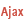HelpWLanguageWLanguage functionsStandard functionsNumeric values management functionsMatrix functionsMatDeletePresentationExampleExistence of the matrix to deleteRelated examplesSee alsoMatAdd (Example)MatAddMatCopy (Example)MatCopyMatCreate (Example)MatCreateMatDelete (Example)MatDeleteMatDeterminant (Example)MatDeterminantMatError (Example)MatErrorMatExist (Example)MatExistMatFill (Example)MatFillMatFloatAdd (Example)MatFloatAddMatFloatMultiply (Example)MatFloatMultiplyMatInvert (Example)MatInvertMatMultiply (Example)MatMultiplyMatNbColumn (Example)MatNbColumnMatNbLine (Example)MatNbLineMatRead (Example)MatReadMatReadColumn (Example)MatReadColumnMatReadLine (Example)MatReadLineMatStack (Example)MatStackMatTranspose (Example)MatTransposeMatWrite (Example)MatWriteMatrix management functionsThe matrices
• Existence of the matrix to delete
WINDEVWEBDEVWINDEV MobileOthersMatDelete (Function)
In french: MatSupprimeNot available
Deletes an existing matrix. The deletion of an unused (or null) matrix from an application is used to free the memory footprint occupied by this matrix.
Remark: All the matrices used in an application are automatically deleted when closing the application.
Example
// Delete a matrix
MatDelete("MyMatrix")
Syntax
MatDelete(<Matrix>)
<Matrix>: Character string
Name of the matrix to delete, defined by MatCreate.
Remarks

Existence of the matrix to delete

The matrix existence is not checked. To check the matrix existence, use MatExist. If the matrix does not exist, MatDelete has no effect.
Related Examples:Unit examples (WINDEV): The Matrix functions [ + ] Using matrices with the WLanguage functions.This example, powered by WINDEV, is used to perform calculations on matrices: addition, multiplication, transposition, inversion...Unit examples (WINDEV Mobile): The Matrix functions [ + ] Using matrices with the WLanguage functions: addition, multiplication, transposition, inversion...
Business / UI classification: Neutral code
Component: wd270mat.dll
Minimum version required
• Version 9